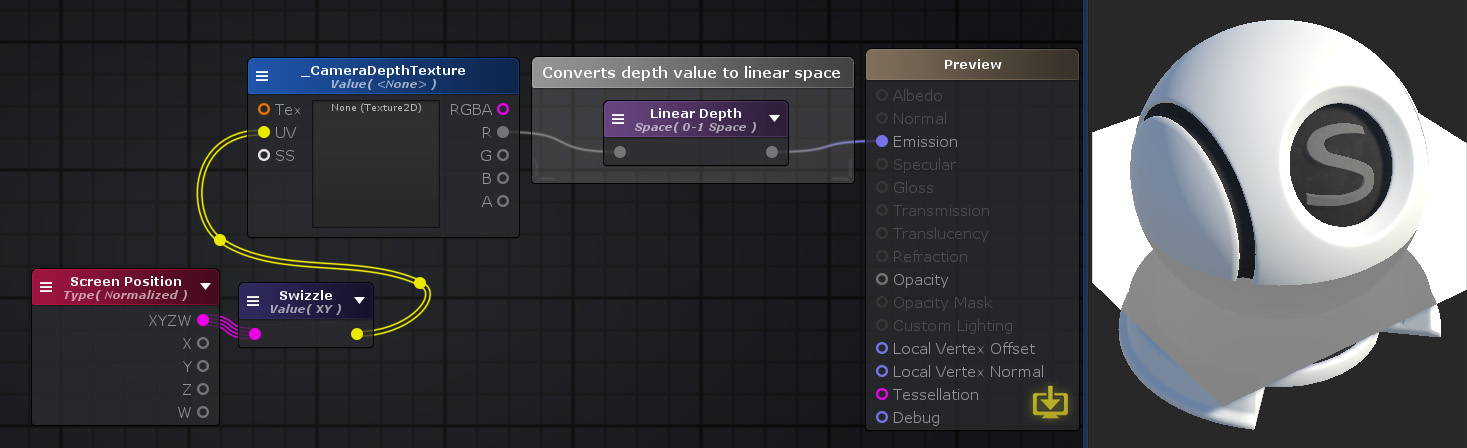Linear Depth Node

The Linear Depth node calculates a linear value, usually given from a depth texture. Values read from a read texture range between 0 and 1, with a non-linear/logarithmic distribution and must be converted to a linear space to be properly used.Nodes used: Screen Position, Swizzle, Texture Sample, Linear Depth

Node Parameter Description Default Value
View Space Linear space for the value to be converted to.
• Eye Space: Returns linear depth on a range between camera's near and far plane
• 0-1 Space: Returns linear depth on a range between 0 and 1
Eye Space

Input Port Description Type
Input Non-linear depth value to be converted. Float